# Supervised Learning

In this chapter, we are going to discuss Supervised Learning. I think this is the most common machine learning algorithm. I would start with an example and hope it will be cleared later. Let’s say we are going to predict housing prices. I take this picture example from Andrew Ng Coursera.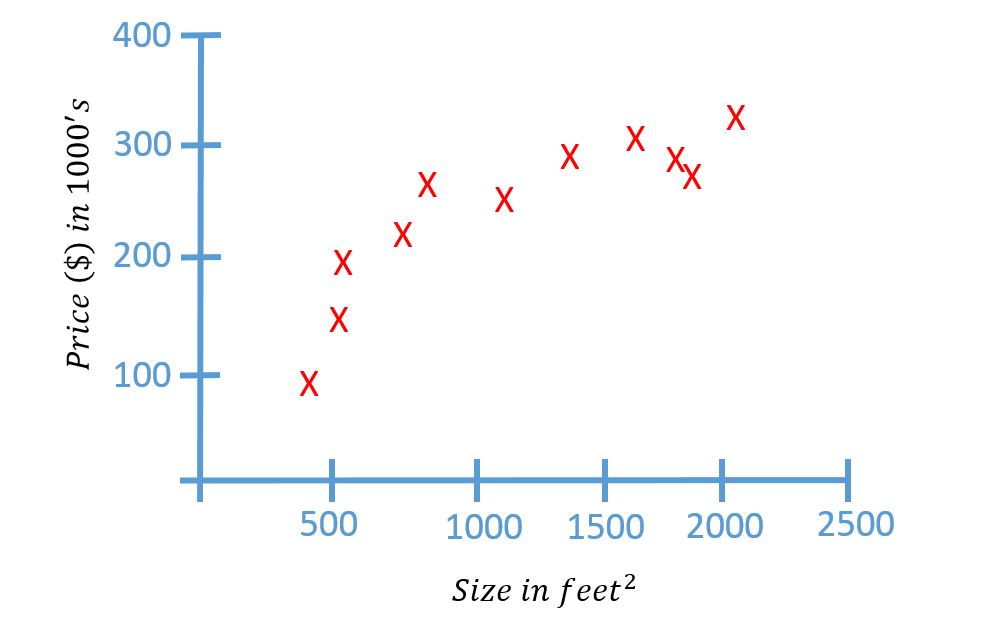Here on the horizontal axes is the size of different houses and on the vertical axis, the price of different houses.

Given this data, let’s say I have a friend who owns $$750 feet^2$$ and He wants to sell the house and I want to know how much I can get for the house. So how the learning algorithm help me in this case? The learning algorithm probably can come out with a straight line through the data and based on that, it looks like maybe the house can be sold for $150,000. But this is not the only output (model) learning algorithm can give.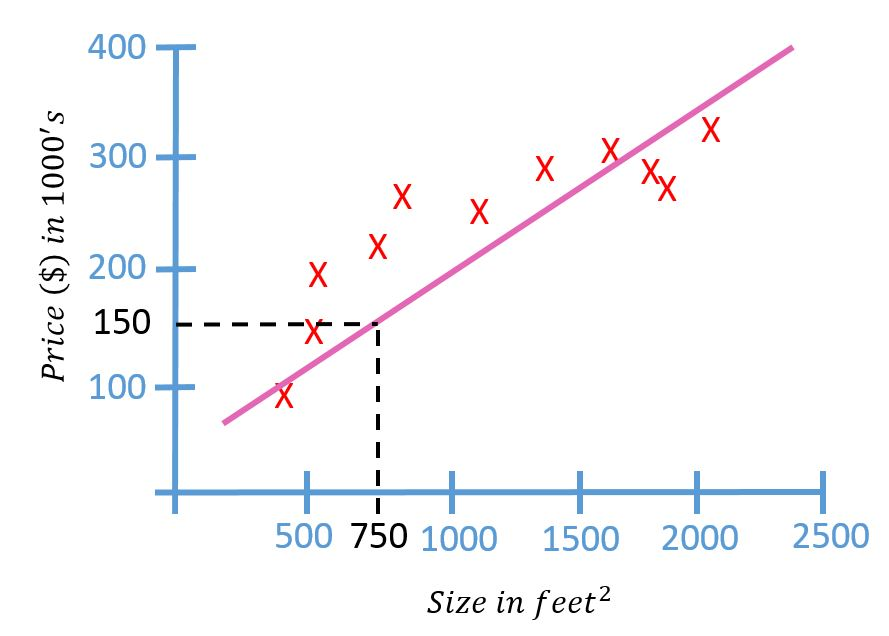There might be a better one. for example, instead of the straight line to the data, we can use a quadratic function (second-order polynomial) to fit the data. And probably the result look like this picture below.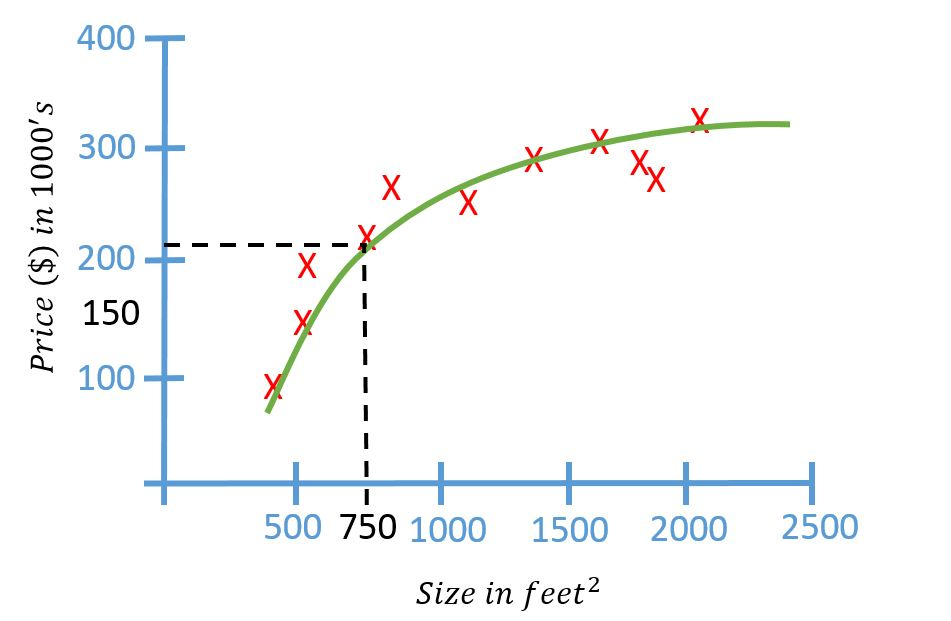The prediction is slightly different with the straight line. In here the house’s price around$200,000. one thing we are going to discuss later is how to decide, do we want to fit a straight line to the data or use a quadratic function.
This is an example of a supervised learning algorithm. The term “supervised learning” refers to the fact that we gave the algorithm a data set, in which the “right answers” (later we call label/ground truth) were given. Remember, We gave dataset of houses in which for every example in this data set, we told it what is the right price that the house sold for. The purpose of the algorithm was to just produce more of these right answers such as for my friend house ($$750 feet^2$$, prediction $200,000). In Machine Learning, this is also called a regression problem. In Regression problem, we are trying to predict a continuous value output. in this case the price. In the previous case, we only use one feature or one attribute. But actually, it can be more than one. Let’s say we have price and size of the houses. we are going to make a system that will decide whether we will buy or not. In that case, maybe our data set will look like this.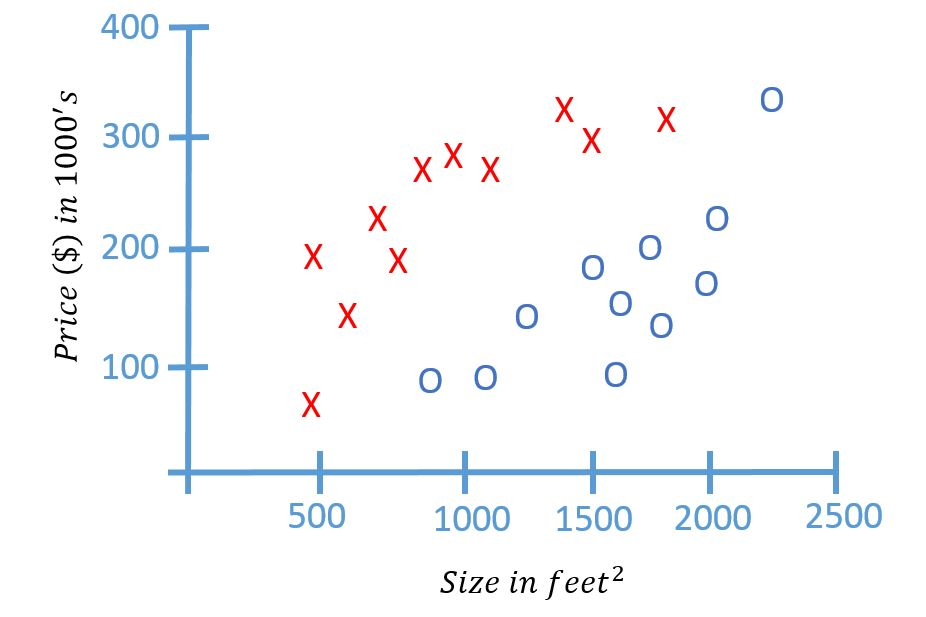In this data set the red cross means data price and size of the houses that we are not going to buy and the blue circle means, the houses that we are going to buy. By given the dataset like this, what the learning algorithm might do us throw the straight line through the data to try to separate out. For example the algorithm with throw the straight line like this picture to separate out whether to buy or not.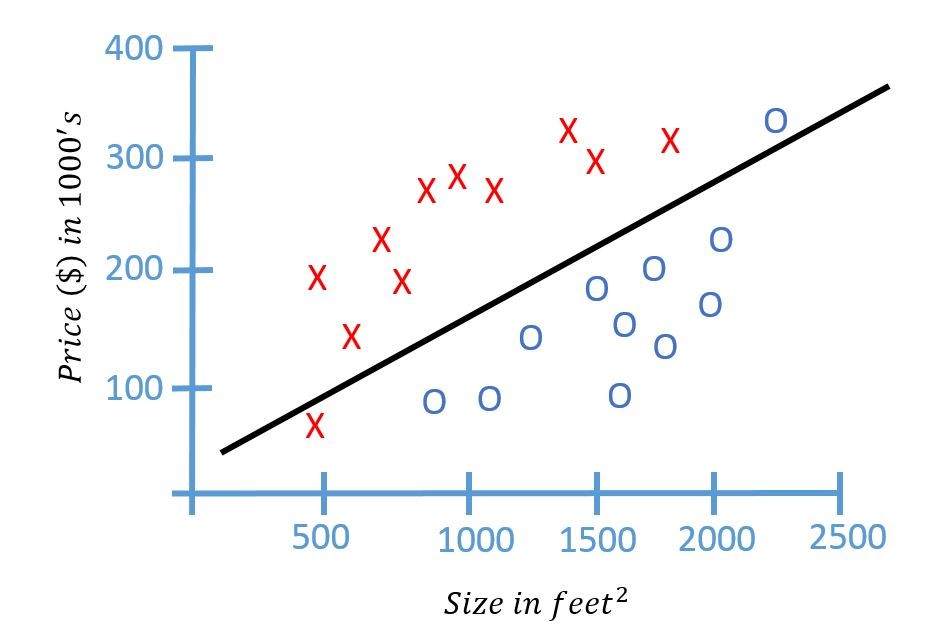Then, if there is a new house that is offered for sale, we can plot the new house into our model. let’s say the house is$200,000 for $$1200 feet^2$$. if we plot the data it would be like this,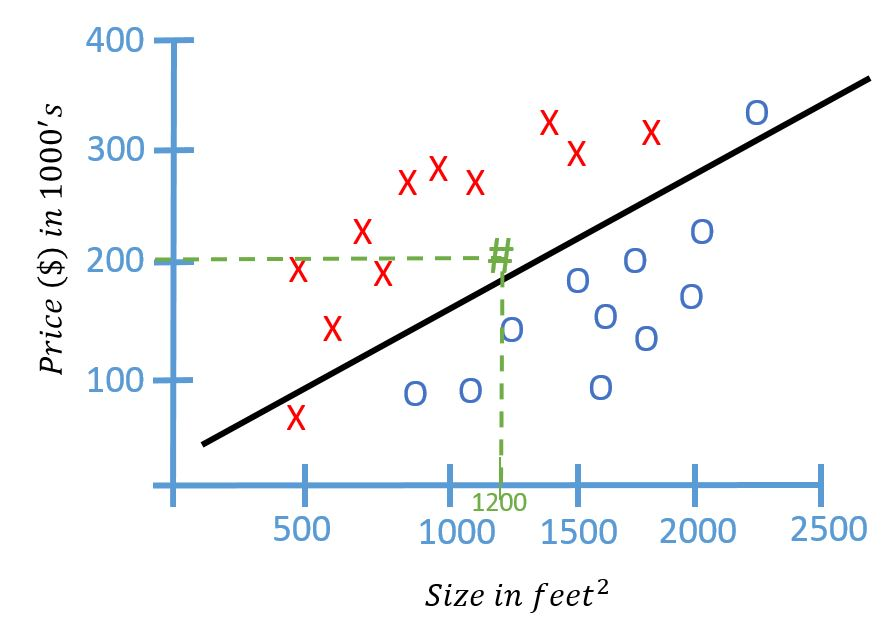By this result, very likely we are not going to buy this house. In this example, we had two features. namely the price and the size, of the house. In other machine learning problem, we will often have more features. This particular example problem is called classification problem (binary classification for a specific name).